Draw Flattened Pixmap VI

LabVIEW 2018 Help

Edition Date: March 2018
Part Number: 371361R-01
View Product InfoLabVIEW 2016 HelpLabVIEW 2017 HelpLabVIEW 2018 HelpLabVIEW 2019 HelpLabVIEW 2020 Help

Owning Palette: Picture Functions VIs

Requires: Base Development System

Draws a 1-, 4-, or 8-bit pixmap or a 24-bit RGB pixmap into a picture.

This VI takes a 1D array of bytes as input and assumes the user completes all packing and padding.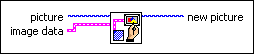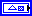picture is the picture to which you want to add the array of data. The default is an empty picture.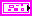image data describes the image you want to draw or manipulate.image type is reserved for future use.image depth specifies the color depth of the image, which is the number of bits to use to describe the color of each pixel in the image. Valid values include 1, 4, 8, and 24 bits per pixel. image depth affects how LabVIEW interprets the values of image and colors.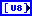image is an array of bytes that describes the color of each pixel in the image in raster order. The value of image depth determines how LabVIEW interprets the value of this input. If image depth is 24, each pixel has three bytes to describe its color. The first byte for each pixel describes the red value, the second byte describes the green value, and the third byte describes the blue value.

If image depth is 8, each pixel has one byte to describe its color. The value of each byte corresponds to an element in colors, which stores 32-bit RGB values where the most-significant byte is zero, followed in order by red, green, and blue values. Valid values include 0 through 255.

If image depth is 4, the behavior is similar to when image depth is 8 except valid values in image include 0 through 15.

If image depth is 1, any value of zero in image corresponds to element 0 in colors. All other values correspond to element 1 in colors.mask is an array of bytes in which each bit describes mask information for a pixel. The first byte describes the first eight pixels, the second byte describes the next eight pixels, and so on. If a bit is zero, LabVIEW draws the corresponding pixel as transparent. If the array is empty, LabVIEW draws all pixels without transparency. If the array does not contain a bit for each pixel in the image, LabVIEW draws any pixels missing from the array without transparency.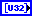colors is an array of RGB color values that correspond to the values in image. The value of image depth determines how LabVIEW interprets the value of this input. colors stores 32-bit RGB values where the most-significant byte is zero, followed in order by red, green, and blue values. Valid values include 0 through 255.

If image depth is 24, LabVIEW ignores this input.

If image depth is 8, the array can have 256 elements.

If image depth is 4, the array can have 16 elements.

If image depth is 1, the array can have 2 elements.Rectangle is a cluster that contains coordinates that describe the bounding rectangle in which you want to draw the image. The VI clips the image to the width and height of the rectangle. The bottom and right edges of the rectangle bounds do not contain image pixels. Horizontal coordinates increase to the right, and vertical coordinates increase to the bottom.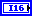left is the horizontal coordinate of the left edge of the rectangle.top is the vertical coordinate of the top edge of the rectangle.right is the horizontal coordinate of the right edge of the rectangle.bottom is the vertical coordinate of the bottom edge of the rectangle.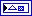new picture is the picture that contains the new image. You can wire this output to any other picture input to add more drawing instructions to the picture.

Draw Flattened Pixmap DetailsNote  This VI interprets 32-bit images as 24-bit images.

You can use this VI with one of the Graphics Formats VIs that reads graphics files in a flattened format. You also can use the Flatten Pixmap VI to produce flattened pixmap data. Use the Unflatten Pixmap VI to unflatten data for use with one of the other VIs used to draw a pixmap.

The Draw Flattened Pixmap VI expects all images to be padded with a 2-byte boundary. The Draw Flattened Pixmap VI does not pad images. The various Graphics Formats VIs which load images also pad images appropriately for use in the Draw Flattened Pixmap VI. If you choose to upload your image in a different way, you need to pad the data with a 2-byte boundary yourself before using the Draw Flattened Pixmap VI.